Скачать презентацию Topic 1 a Review of Consumer Theory demand Review

19704d4490bf7ef95b48cb03e11b1794.ppt

• Количество слайдов: 11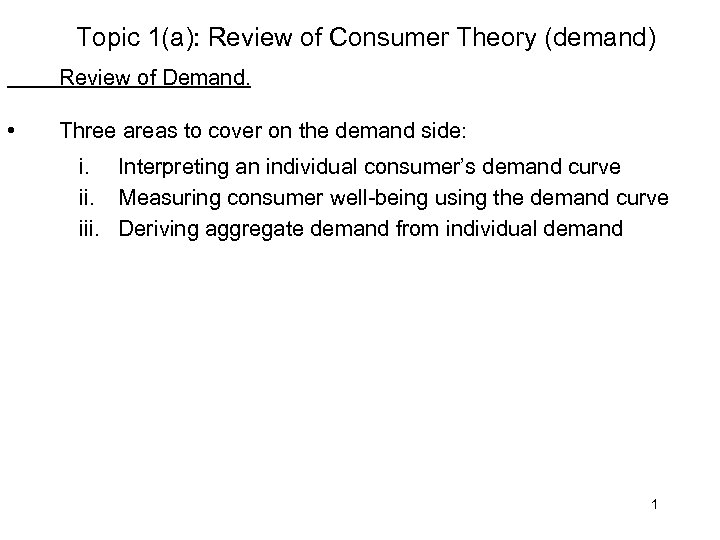Topic 1(a): Review of Consumer Theory (demand) Review of Demand. • Three areas to cover on the demand side: i. Interpreting an individual consumer’s demand curve ii. Measuring consumer well-being using the demand curve iii. Deriving aggregate demand from individual demand 1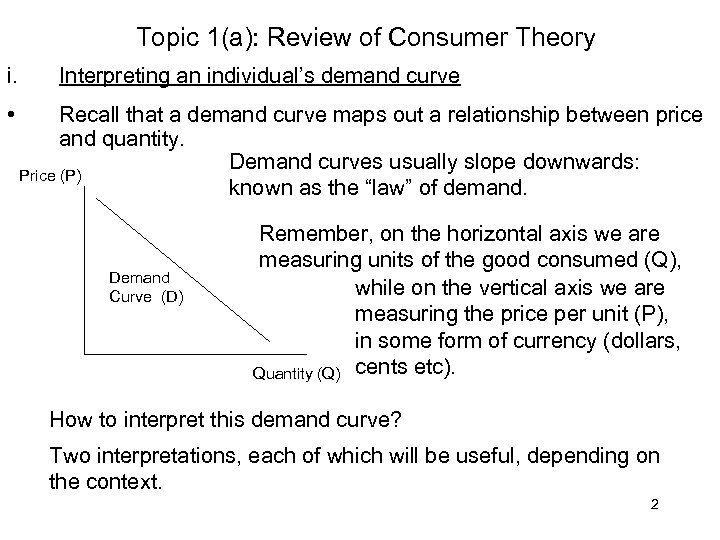Topic 1(a): Review of Consumer Theory i. • Interpreting an individual’s demand curve Recall that a demand curve maps out a relationship between price and quantity. Demand curves usually slope downwards: Price (P) known as the “law” of demand. Demand Curve (D) Remember, on the horizontal axis we are measuring units of the good consumed (Q), while on the vertical axis we are measuring the price per unit (P), in some form of currency (dollars, Quantity (Q) cents etc). How to interpret this demand curve? Two interpretations, each of which will be useful, depending on the context. 2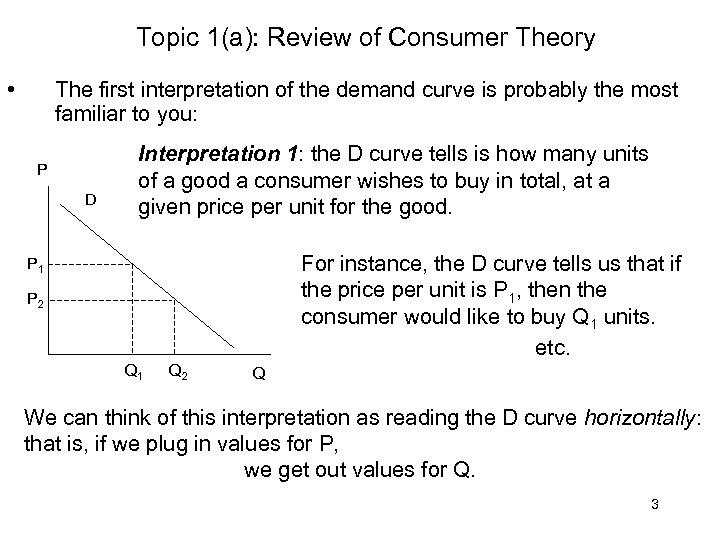Topic 1(a): Review of Consumer Theory • The first interpretation of the demand curve is probably the most familiar to you: P D Interpretation 1: the D curve tells is how many units of a good a consumer wishes to buy in total, at a given price per unit for the good. For instance, the D curve tells us that if the price per unit is P 1, then the consumer would like to buy Q 1 units. etc. P 1 P 2 Q 1 Q 2 Q We can think of this interpretation as reading the D curve horizontally: that is, if we plug in values for P, we get out values for Q. 3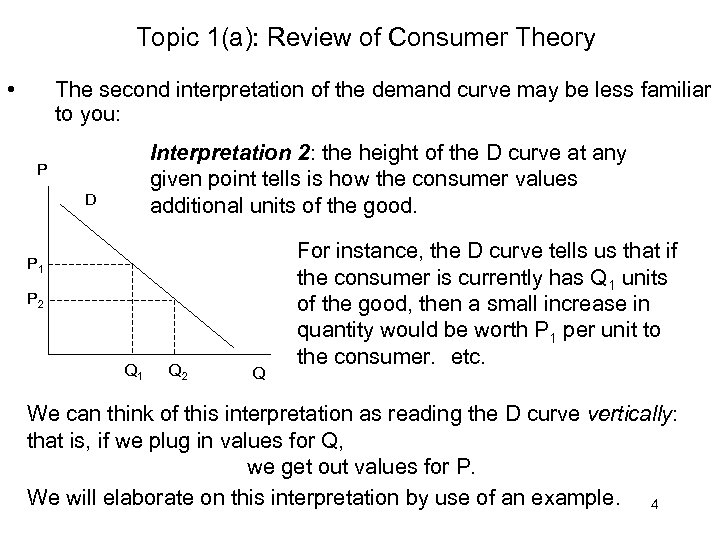Topic 1(a): Review of Consumer Theory • The second interpretation of the demand curve may be less familiar to you: Interpretation 2: the height of the D curve at any given point tells is how the consumer values additional units of the good. P D P 1 P 2 Q 1 Q 2 Q For instance, the D curve tells us that if the consumer is currently has Q 1 units of the good, then a small increase in quantity would be worth P 1 per unit to the consumer. etc. We can think of this interpretation as reading the D curve vertically: that is, if we plug in values for Q, we get out values for P. We will elaborate on this interpretation by use of an example. 4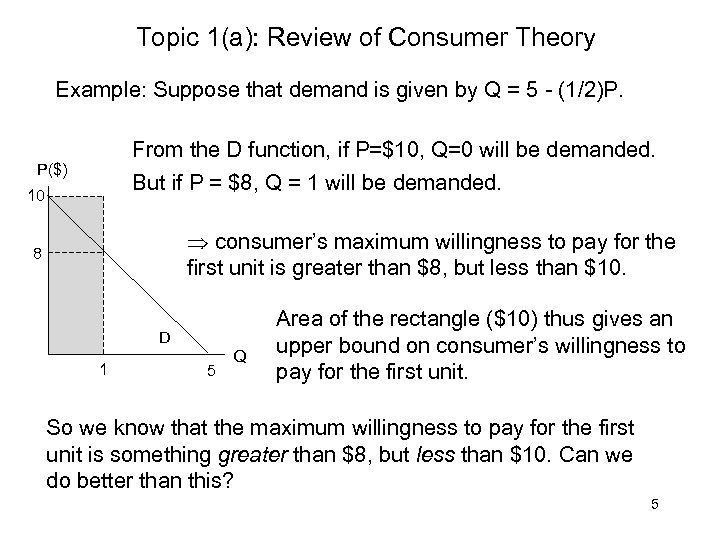Topic 1(a): Review of Consumer Theory Example: Suppose that demand is given by Q = 5 - (1/2)P. From the D function, if P=\$10, Q=0 will be demanded. P(\$) But if P = \$8, Q = 1 will be demanded. 10 consumer’s maximum willingness to pay for the first unit is greater than \$8, but less than \$10. 8 D 1 5 Q Area of the rectangle (\$10) thus gives an upper bound on consumer’s willingness to pay for the first unit. So we know that the maximum willingness to pay for the first unit is something greater than \$8, but less than \$10. Can we do better than this? 5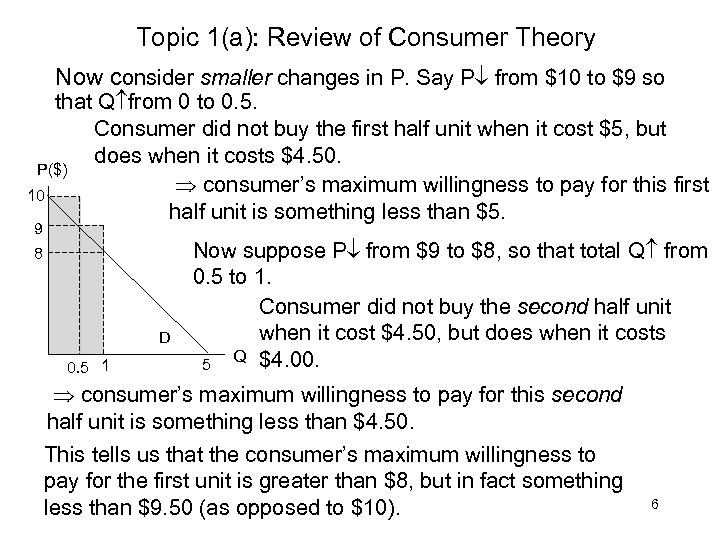Topic 1(a): Review of Consumer Theory Now consider smaller changes in P. Say P from \$10 to \$9 so that Q from 0 to 0. 5. Consumer did not buy the first half unit when it cost \$5, but does when it costs \$4. 50. P(\$) consumer’s maximum willingness to pay for this first 10 half unit is something less than \$5. 9 8 D 0. 5 1 Now suppose P from \$9 to \$8, so that total Q from 0. 5 to 1. Consumer did not buy the second half unit when it cost \$4. 50, but does when it costs 5 Q \$4. 00. consumer’s maximum willingness to pay for this second half unit is something less than \$4. 50. This tells us that the consumer’s maximum willingness to pay for the first unit is greater than \$8, but in fact something less than \$9. 50 (as opposed to \$10). 6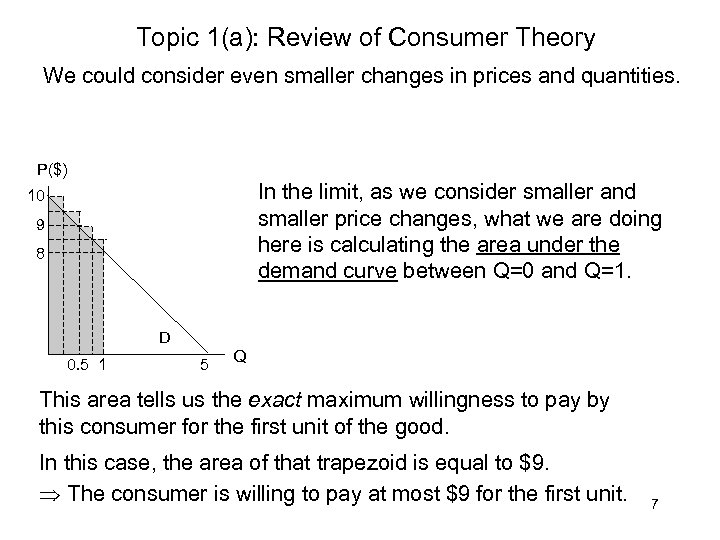Topic 1(a): Review of Consumer Theory We could consider even smaller changes in prices and quantities. P(\$) In the limit, as we consider smaller and smaller price changes, what we are doing here is calculating the area under the demand curve between Q=0 and Q=1. 10 9 8 D 0. 5 1 5 Q This area tells us the exact maximum willingness to pay by this consumer for the first unit of the good. In this case, the area of that trapezoid is equal to \$9. The consumer is willing to pay at most \$9 for the first unit. 7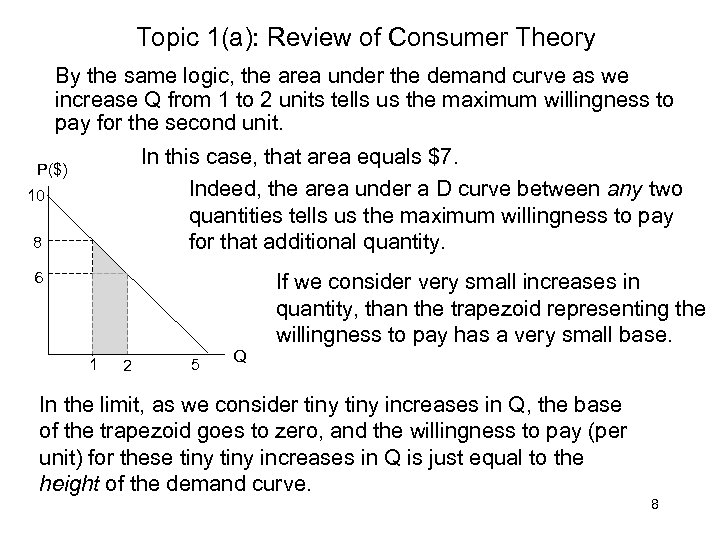Topic 1(a): Review of Consumer Theory By the same logic, the area under the demand curve as we increase Q from 1 to 2 units tells us the maximum willingness to pay for the second unit. In this case, that area equals \$7. Indeed, the area under a D curve between any two quantities tells us the maximum willingness to pay for that additional quantity. P(\$) 10 8 6 If we consider very small increases in quantity, than the trapezoid representing the willingness to pay has a very small base. 1 2 5 Q In the limit, as we consider tiny increases in Q, the base of the trapezoid goes to zero, and the willingness to pay (per unit) for these tiny increases in Q is just equal to the height of the demand curve. 8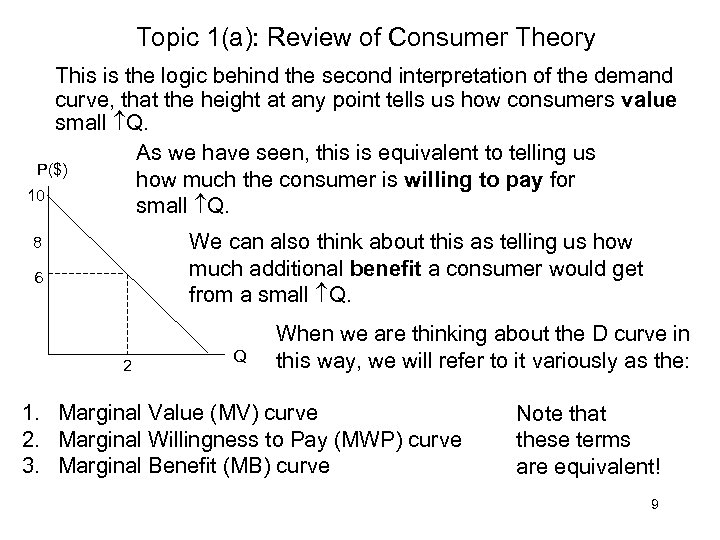Topic 1(a): Review of Consumer Theory This is the logic behind the second interpretation of the demand curve, that the height at any point tells us how consumers value small Q. As we have seen, this is equivalent to telling us P(\$) how much the consumer is willing to pay for 10 small Q. We can also think about this as telling us how much additional benefit a consumer would get from a small Q. 8 6 2 Q When we are thinking about the D curve in this way, we will refer to it variously as the: 1. Marginal Value (MV) curve 2. Marginal Willingness to Pay (MWP) curve 3. Marginal Benefit (MB) curve Note that these terms are equivalent! 9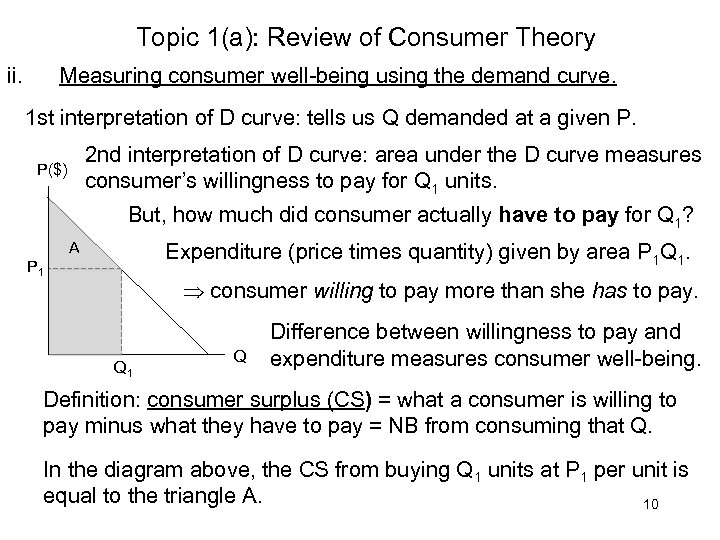Topic 1(a): Review of Consumer Theory ii. Measuring consumer well-being using the demand curve. 1 st interpretation of D curve: tells us Q demanded at a given P. 2 nd interpretation of D curve: area under the D curve measures consumer’s willingness to pay for Q 1 units. P(\$) But, how much did consumer actually have to pay for Q 1? A Expenditure (price times quantity) given by area P 1 Q 1. P 1 consumer willing to pay more than she has to pay. Q 1 Q Difference between willingness to pay and expenditure measures consumer well-being. Definition: consumer surplus (CS) = what a consumer is willing to pay minus what they have to pay = NB from consuming that Q. In the diagram above, the CS from buying Q 1 units at P 1 per unit is equal to the triangle A. 10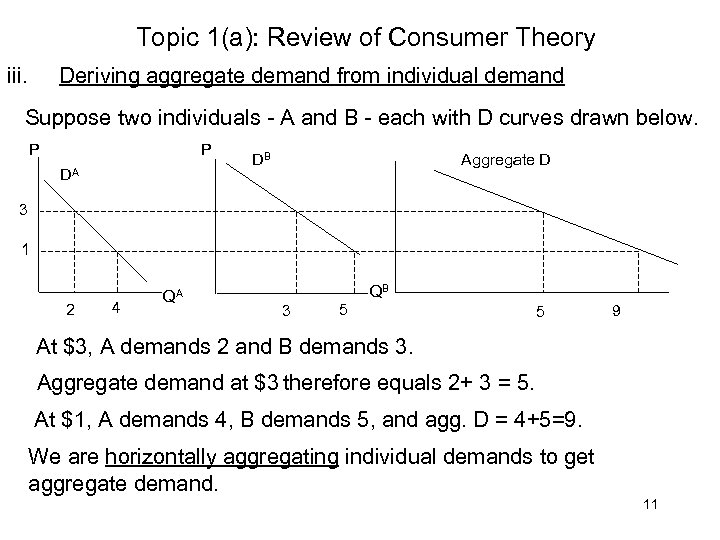Topic 1(a): Review of Consumer Theory iii. Deriving aggregate demand from individual demand Suppose two individuals - A and B - each with D curves drawn below. P P DA Aggregate D DB 3 1 2 4 QA QB 3 5 5 9 At \$3, A demands 2 and B demands 3. Aggregate demand at \$3 therefore equals 2+ 3 = 5. At \$1, A demands 4, B demands 5, and agg. D = 4+5=9. We are horizontally aggregating individual demands to get aggregate demand. 11